# #1. Numbers¶

Note

If you have any ideas or enhancements for this page, please edit it on GitHub!

Following documentation is a cooperative result combined from our Discord chat and numerous pull requests. Thanks to everyone who helped!

Download radio transmission recording. It was originally received at ~5 GHz and scaled down to ~500 Hz to make signal audible for humans.

## Spectrogram¶

Spectrogram of the recording, rendered with a notebook by Discord user @nya: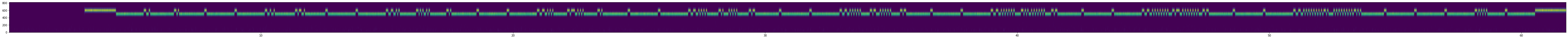## Image¶

A 2D image created by:

1. Converting low and high frequency spans into black and white squares respectively.

2. Rearranging these squares into a rectangle instead of a single line.

Contributed by Discord user @elventian.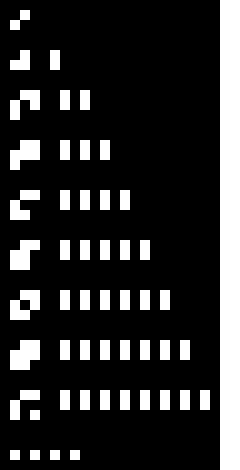## Interpretation¶

Based on the discussions with Discord users @nya, @Kilew, @fryguybob, @aaaa1, @gltronred and @elventian.

Probably the symbols on the left represent numbers and the number of elements on the right is the unary representation of this number.

Symbols on the left look like a binary encoding that should work for numbers 1..15. Picture says 8, because we have hard data only up to 8: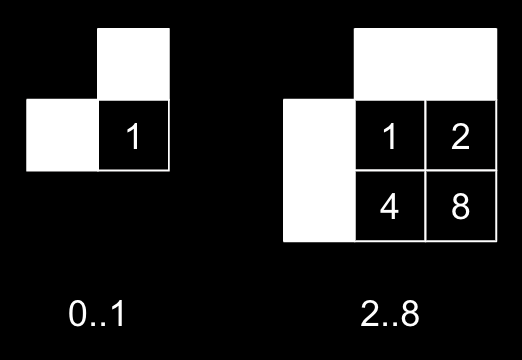According to this theory we can speculate that the numbers 9..15 would be represented with these symbols: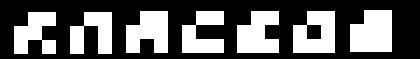Based on this logic the symbols could be extended further like this: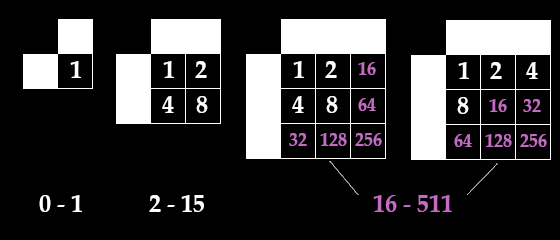Second transmission seems to support the second conjecture (right picture).

## Code¶

This Rust code generates decoded images similar to the image included above from WAV files.

Contributed by Discord user @aaaa1.

Example output: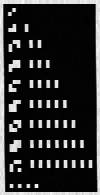// [dependencies]
// png = "0.16"
// hound = "3.4"

fn main() {
let spec = r.spec();
let samples: Vec<i16> = r.into_samples().map(Result::unwrap).collect();

let freq = 600;
let step = 2.0 * std::f32::consts::PI * freq as f32 / spec.sample_rate as f32;
let xys: Vec<(f32, f32)> = samples.iter().copied().enumerate().map(|(i, s)| {
let s = s as f32;
let a = i as f32 * step;
(a.cos() * s, a.sin() * s)
}).collect();

let mut axyz = vec![(0.0, 0.0)];
for (x, y) in xys {
let last = *axyz.last().unwrap();
axyz.push((last.0 + x, last.1 + y));
}

let mut ds: Vec<f32> = axyz.iter().zip(axyz.iter().skip(1000)).map(|(xy1, xy2)| {
let dx = xy1.0 - xy2.0;
let dy = xy1.1 - xy2.1;
dx * dx + dy * dy
}).collect();
let max = *ds.iter().max_by(|x, y| x.partial_cmp(y).unwrap()).unwrap();
ds.iter_mut().for_each(|x| *x /= max);

let width = 100usize;
let height = 195usize;

let w = std::fs::File::create("res.png").unwrap();
let w = std::io::BufWriter::new(w);
let mut encoder = png::Encoder::new(w, width as u32, height as u32);
encoder.set_color(png::ColorType::Grayscale);
encoder.set_depth(png::BitDepth::Eight);
let mut w = encoder.write_header().unwrap();

let mut data = vec![0u8; width * height];
for (i, cell) in data.iter_mut().enumerate() {
let x = i % width;
let y = i / width / 4;
*cell = (ds.get((x + y * width) * 529 + 132400).copied().unwrap_or(0.0) * 255.0) as u8;
}
w.write_image_data(&data).unwrap();
}